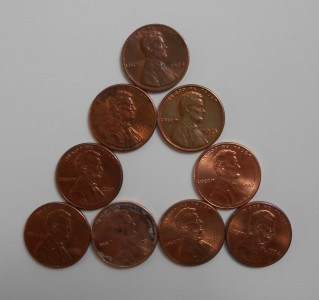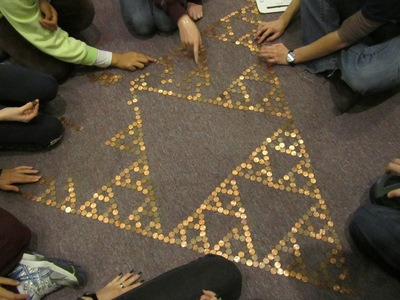### Math Monday: Penny Sierpinsky Triangle

by Glen Whitney

Here’s a project we did a long time ago in collaboration with Vi Hart, that somehow never made it into Math Mondays. The idea is simple: lay out pennies on a large horizontal surface, such as a floor, in the pattern of a Sierpinski triangle. How many? Well, the basic triangle with a one-penny size holerequires nine pennies, and each generation after that requires three times as many pennies, so a sixth-generation triangle requires three to the seventh power pennies, or 2,187 pennies in all (better bring a few extra). In theory, you could just start laying down pennies in the triangular pattern, but in practice you had better start with a string twenty-four feet long marked off in two-foot intervals (if you are making the sixth-generation triangle). Hold the two ends together, get friends to hold on at the eight- and sixteen-foot marks, and stretch the string into an equilateral triangle on the floor. Mark the vertices of the triangle on the floor with tape, and mark the two foot interval points along each edge with tape as well. All of these marks will correspond to the corners of fourth-generation subtriangles. Now start laying the pennies down, starting from these marks as guides. Here is one-third of the final layout in progress:And here is the completed triangle:If you go for the next generation larger, or make other cool Sierpinski triangles or penny layouts, let us know at mondays@momath.org — thanks!